This post will demonstrate how the %FiniteHMM macro can automatically preprocess input data and postprocess output tables for finite Hidden Markov Models (HMMs) by using PROC HMM. First, you will be introduced to finite HMMs models and PROC HMM. Next, a brief description will be given of the %FiniteHMM macro. Finally, a couple of examples will presented on how to use the macro.

## Introducing Finite HMM with PROC HMM

The HMM procedure supports HMMs which have been widely applied in economics, finance, science, and engineering. A finite HMM is one type supported by PROC HMM. In theory, a finite HMM assumes that the response variable is discrete (whether its value is numeric or categorical). PROC HMM assumes that the response variable is composed of consecutive natural numbers starting with 1. However, for most real-world business data, the variable of interest could be recorded in other formats, such as categorical levels, decimals, negative numbers, and so on. If the response variable contains values other than natural numbers, PROC HMM will register errors. In other cases, a response variable could be recorded as a nonconsecutive natural number. It could also be recorded as a consecutive natural number, but not starting from 1. In the last two cases, PROC HMM will not generate an error, but the results will be incorrect. In these cases, PROC HMM still assumes the response variable has consecutive values from 1 to the maximum value of the response variable. Consequently, the output tables will contain unnecessary parameters. Therefore, it is likely that you will need to preprocess the data before using PROC HMM to perform finite HMMs.

For example, if you are interested in modeling a dynamic feature of promotional channels for a buyer, the response variable y1 could be recorded with values such as ‘aa email’, ‘bb mail’, ‘cc phone’, ‘dd flyer’, ‘ee seminar’, and ‘ff forum’. Applying PROC HMM directly on y1 will cause an error. You would need to preprocess the data by creating a consecutively natural-number-valued variable y corresponding to the levels of y1. Then apply PROC HMM on y if you assume that y1 is in the data set finite1. The following SAS program demonstrates the preprocessing:

``` data finite2; set finite1; if y1='aa email' then y=1; if y1='bb mail' then y=2; if y1='cc phone' then y=3; if y1='dd flyer' then y=4; if y1='ee seminar' then y=5; if y1='ff forum' then y=6; run;```

The variable y in the finite2 data set has values of the consecutive natural numbers 1 through 6. After you load the data set finite2 to a defined CAS library mycas, you can use PROC HMM on y for a finite HMM as shown:

``` ods output CPM=my_cpm_0 ParameterEstimates=my_est_0; proc hmm data=mycas.finite2; id time=t section=section; model y / type=finite nstate=3 nCategory=6 method=MAP; estimate out=mycas.est_0 outall=mycas.estall_0; forecast out=mycas.fcst_0 lead=4; run;```

Because y has values 1 through 6, the output tables have information about the levels of y only, not the levels of y1. To understand and analyze the results for the original y1, you need to do postprocessing by manually matching the y levels to the levels of y1. This can be tedious if y1 has too many levels. Hence it would be helpful if there is a tool to automatically handle the preprocessing and post-processing in such cases. SAS macro %FiniteHMM is the tool to do that.

## The SAS macro %FiniteHMM

The %FiniteHMM macro is a wrapper for finite HMMs with PROC HMM.  The macro treats the response variable as a categorical variable. It automatically preprocesses the input data, applies PROC HMM to the internally created response variable, and postprocesses the results in terms of the original variable.

In the preprocessing stage, the macro will:

• Create the internal response variable _mody_ with consecutive natural numbers (starting from 1) as its values
• Update the NCATEGORY= option to the actual number of levels of the original response

Then, it will apply PROC HMM to the internally created response variable for finite HMMs.

In the postprocessing stage, the macro will:

• Update the label of categorical columns with their corresponding levels in the original response variable for the ODS CPM output table and the CAS output forecast table
• Add a column named RespVarLevel that indicates the corresponding levels in the original response variable for both the ODS parameter estimates table and the CAS output parameter estimates table

The parameters of the %FiniteHMM macro are defined to correspond to the statements in PROC HMM. The options in each statement of PROC HMM become the parameter values in the macro. This macro is useful in these three cases:

• Response variable contains values other than natural numbers
• Response variable is composed of nonconsecutive natural numbers
• Response variable is composed of consecutive natural numbers but does not start with 1

## Examples of Using %FiniteHMM

Finally, here are several examples that show how to use the %FiniteHMM macro. First, you need to simulate a data set. You can use the simulation SAS code in examples of %FiniteHMM to simulate a data set finite1. The data set finite1 has three variables: y, t, and section. Among them, y is the response variable with values 1 to 6, t is the time with values 1 to 500, and section is the section variable with values 1 to 3. This SAS code creates three response variables y1, y2, y3 for our examples.

``` data finite2; length y1 \$15.; set finite1; if y=1 then y1='aa email'; if y=2 then y1='bb mail'; if y=3 then y1='cc phone'; if y=4 then y1='dd flyer'; if y=5 then y1='ee seminar'; if y=6 then y1='ff forum'; if y<=3 then y2=2; else y2=6; y3=y+2; run;```

Here in data set finite2 y1 is a categorical variable with values in the set ['aa email', 'bb mail', 'cc phone', 'dd flyer', 'ee seminar’, 'ff forum'], y2 is a natural-number-valued variable with values in the set [2, 6], and y3 has consecutive natural number values in the set [3, 4, 5, 6, 7, 8].

Applying PROC HMM on y1 generates errors since y1 contains values other than natural numbers. There is no error applying PROC HMM on y2 and y3, but the results are not correct. PROC HMM still assumes that y2 has consecutive integer values from 1 to 6, and y3 has consecutive integer values from 1 to 8. So both y2 and y3, the output tables will contain redundant parameters and the results are incorrect. By using %FiniteHMM, you can get the desired results for all three variables.

Assuming that CAS library mycas has been defined, the following SAS code uploads the finite2 data set to the default library of your current active CAS session:

``` proc casutil; load data=finite2 casout='finite2' replace; run; quit;```

### Example 1: Applying %FiniteHMM on the Categorical Response y1

To apply %FiniteHMM on y1, you can use this SAS code:

``` %FiniteHMM( data = %str(mycas.finite2), proc = %str(labelSwitch=(sort=asc(cpm))), id = %str(time=t section=section), model = %str(y1 / type=finite nstate=3 nCategory=6 method=MAP), estimate = %str(out=mycas.est_1 outall=mycas.estall_1), forecast = %str(out=mycas.fcst_1 lead=4), odsout = %str(CPM=my_cpm_1 ParameterEstimates=my_est_1));```

You can then print out the ODS table my_cmp_1, ODS table my_est_1, and CAS output table mycas.fcst_1. The labels of Category1 to Category6 in the CPM and forecast table are linked to the levels of response variable y1. The column RespVarLevel in the parameter estimates table shows the levels of y1.

``` proc print data=my_cpm_1 label noobs; run;   proc print data=my_est_1 noobs; run;   proc print data=mycas.fcst_1 label noobs; run;```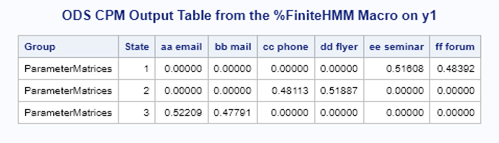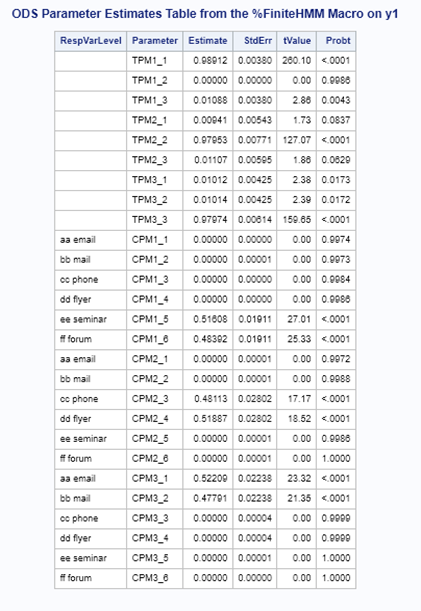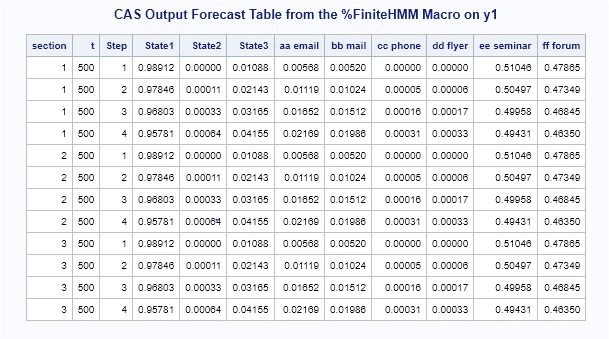### Example 2: Applying %FiniteHMM on the Non-consecutive Natural-Number-Valued Response y2

To apply %FiniteHMM on y2, you can use this SAS code:

``` %FiniteHMM( data = %str(mycas.finite2), proc = %str(labelSwitch=(sort=asc(cpm))), id = %str(time=t section=section), model = %str(y2 / type=finite nstate=3 nCategory=6 method=MAP), estimate = %str(out=mycas.est_2 outall=mycas.estall_2), forecast = %str(out=mycas.fcst_2 lead=4), odsout = %str(CPM=my_cpm_2 ParameterEstimates=my_est_2));```

Similarly, you can then print out the ODS table my_cmp_2, ODS table my_est_2, and CAS output table mycas.fcst_2.

``` proc print data=my_cpm_2 label noobs; run;   proc print data=my_est_2 noobs; run;   proc print data=mycas.fcst_2 label noobs; run;```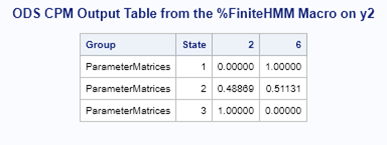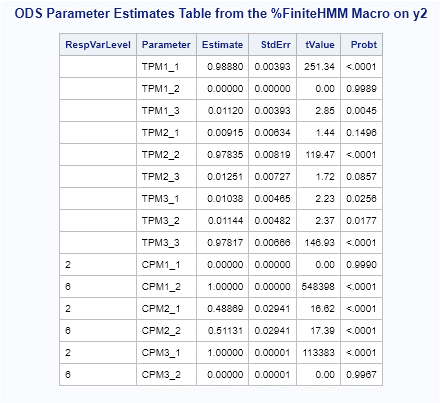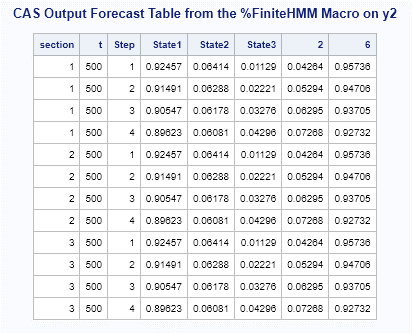### Example 3: Using %FiniteHMM when the Response Variable y3 Has Consecutive Natural Number Values but not Starting from 1

To apply %FiniteHMM on y3, you can use the SAS code:

``` %FiniteHMM( data = %str(mycas.finite2), proc = %str(labelSwitch=(sort=asc(cpm))), id = %str(time=t section=section), model = %str(y3 / type=finite nstate=3 nCategory=6 method=MAP), estimate = %str(out=mycas.est_3 outall=mycas.estall_3), forecast = %str(out=mycas.fcst_3 lead=4), odsout = %str(CPM=my_cpm_3 ParameterEstimates=my_est_3));```

You can then print out the ODS table my_cmp_3, ODS table my_est_3, and CAS output table mycas.fcst_3.

``` proc print data=my_cpm_3 label noobs; run;   proc print data=my_est_3 noobs; run;   proc print data=mycas.fcst_3 label noobs; run;```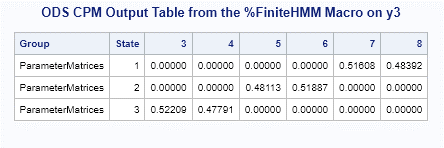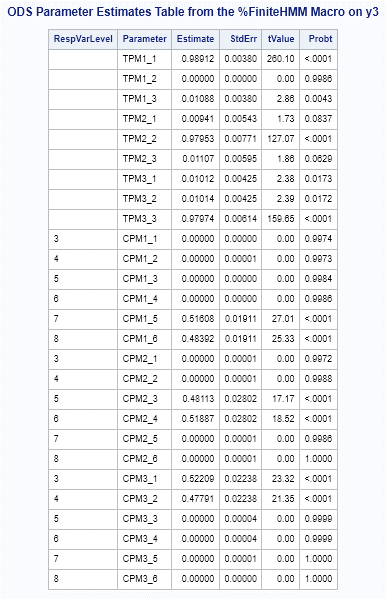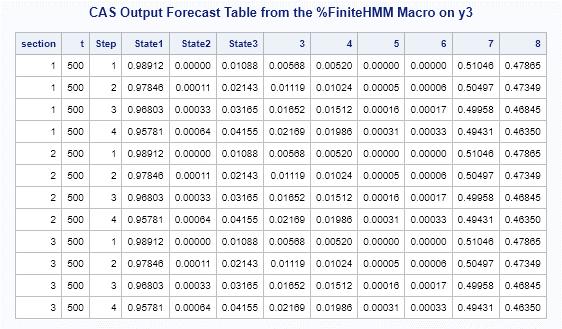Three examples of using %FiniteHMM have been presented. In each, when directly applying PROC HMM for a finite HMM, error messages are generated or the results are incorrect because of the unnecessary parameters in output tables. Applying %FiniteHMM generates the correct results for all these cases.

## Summary

When you apply PROC HMM to a finite HMM, PROC HMM expects that the response variable is composed of consecutive natural numbers starting from 1. If the values of the response variable in the input data set are not composed of consecutive natural numbers starting from 1, you might get errors or incorrect results. In such cases, the %FiniteHMM macro helps to automate the preprocessing and postprocessing. When you apply %FiniteHMM, the ODS output table of CPM and CAS output table of forecasts have labels that link to the levels of the original response variable. The ODS output table and CAS output table of parameter estimates have a column called RespVarLevel. The RespVarlevel column contains values that link to the levels of the original response variable. Hence these tables are ready for analysis on the original response variable.

%FiniteHMM treats the response variable as a categorical variable. This is consistent with the theoretical assumption of finite HMM. Using %FiniteHMM can save you a lot of time when you have a big data set, when the response variable has many levels of values, or when you are building many models.

Share